# Bear Call Spread Payoff, Break-Even and R/R

This page explains bear call spread profit and loss at expiration and the calculation of its maximum gain, maximum loss, break-even point and risk-reward ratio.

## Bear Call Spread Basic Characteristics

Bear call spread, also known as short call spread, is a bearish option strategy using two call options – one short call with a lower strike and one long call with a higher strike. It has limited risk and limited possible profit. This strategy is typically used when a trader expects the underlying security to go down, but only moderately.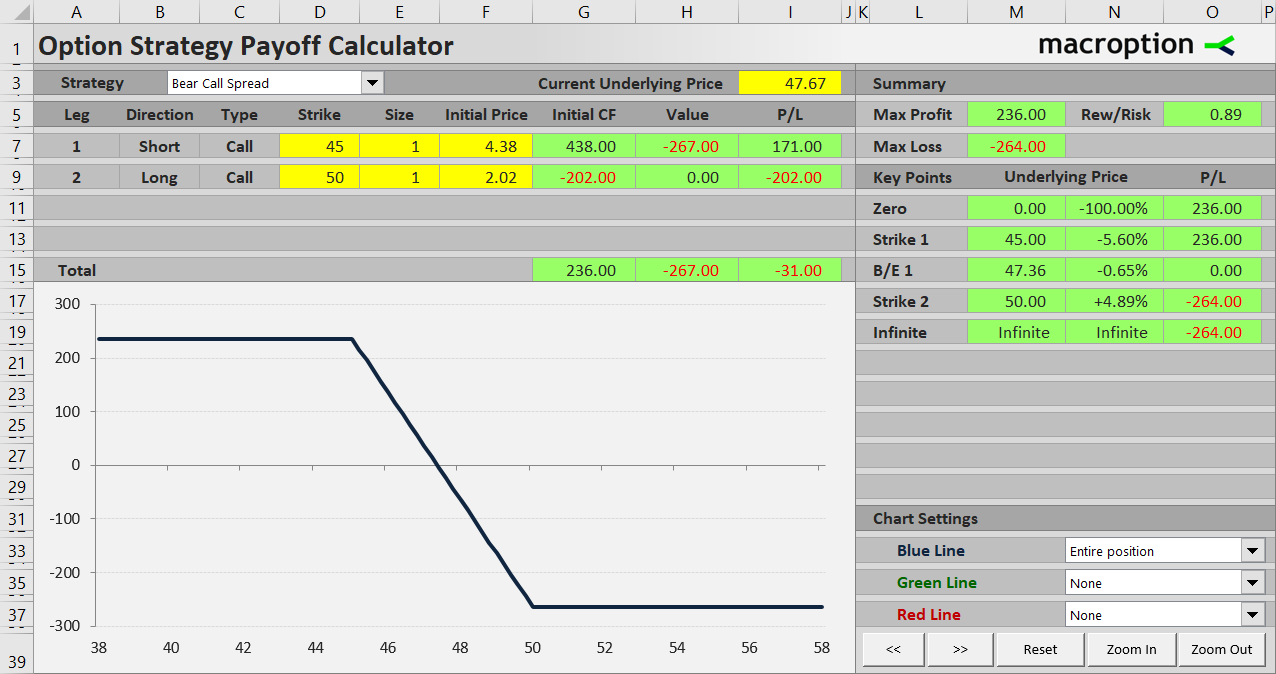Bear call spread is the inverse of bull call spread. Besides the direction of underlying price exposure the main difference is that it is a credit spread – initial cash flow is positive when opening a bear call spread position, because price of the lower strike call being sold is typically higher than price of the higher strike call being bought. The objective of the trade is to defend the net premium received.

Let's have a look at the profit and loss profile and possible scenarios using an example.

Consider a position created by the following two transactions:

Buy one contract of a \$50 strike call option for \$2.02 per share.

Sell one contract of a \$45 strike call, on the same underlying, with the same expiration date, for \$4.38 per share.

For the \$45 strike call we receive \$438 (assuming US equity option contracts, which represent 100 shares). For the \$50 strike call we pay \$202. The difference (\$236) is net premium received, or the positive initial cash flow from the trade.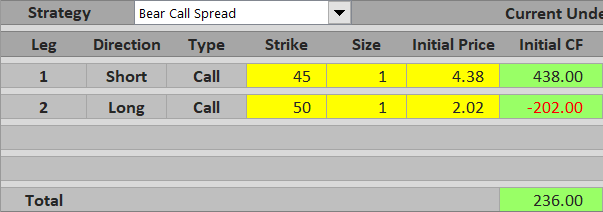If you have seen the bull call spread example, you may have noticed that this trade is the exact opposite of that – we are using the same example, only now we are taking the other side of the trade.

If we hold the position until expiration, there are three possible scenarios. Let's have a look at each in more detail.

## Scenario 1 (Maximum Loss)

With a bear call spread you want the underlying price to go down. The worst case scenario is obviously when you are wrong about the direction and underlying price ends up substantially higher by the time your options expire. Using our example, let's say the underlying gets to \$52 – above both strikes.

Both calls are in the money. You are short the lower strike (\$45) call and lose \$52 minus \$45 = \$7 per share or \$700 for one contract. However, this loss is partly offset with the long \$50 call, which makes \$200 (\$52 – \$50 = \$2 per share). In fact, regardless of how high the underlying price will get, further losses from the short call will be exactly offset by further gains from the long call. For any underlying price at or above \$50 your total loss from the trade will always be \$500 (\$5 per share = difference between the two strikes) minus net premium received when opening the position (\$236), which is \$264. This amount matches the maximum possible profit from the bull call spread example, which is again the exact other side of this trade.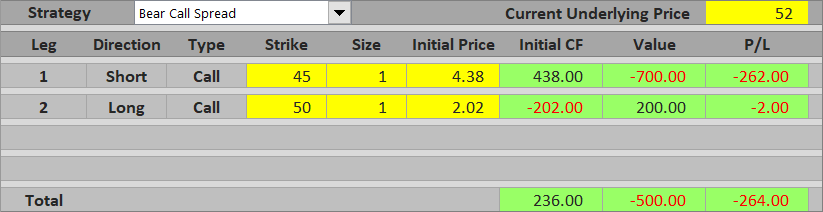Maximum possible loss from a bear call spread equals the difference between strikes minus net premium received. It applies for underlying price exactly at or above the higher strike.

## Scenario 2 (Maximum Profit)

On the other side of the spectrum, if you are right about the direction and underlying price gets below the lower strike at expiration, both options are worthless. There are no further gains or losses at expiration and the trade's total outcome equals the cash you have received when opening the position.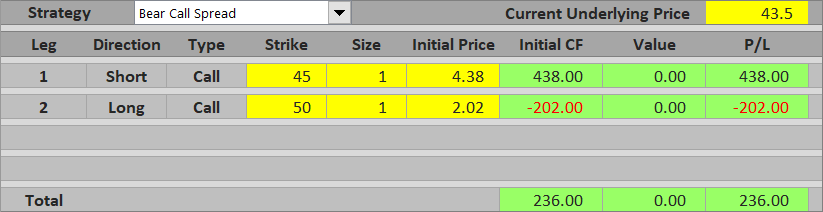Maximum possible profit from a bear call spread trade equals net premium received. It applies when underlying price ends up exactly at or below the lower strike.

## Scenario 3 (Between the Strikes)

If underlying price ends up between the two strikes at expiration, the trade's total profit or loss depends on where exactly it is. Because a bear call spread is a bearish trade, the lower the underlying gets, the better.

For example, with the underlying at \$46, the short \$45 call is in the money by \$1 per share, which means you lose \$100. The long \$50 call is out of the money. Combined with net premium received in the beginning, the total profit from the trade is \$236 – \$100 = \$136.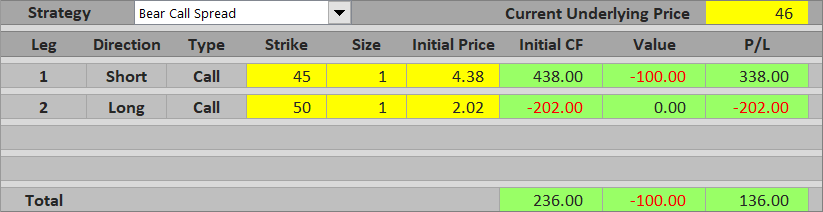On the other hand, if the underlying ends up at \$48.50, the short \$45 is worth \$3.50 per share, or \$350, and wipes out the entire premium received and more. The trade turns to a loss equal to \$236 – \$350 = – \$114.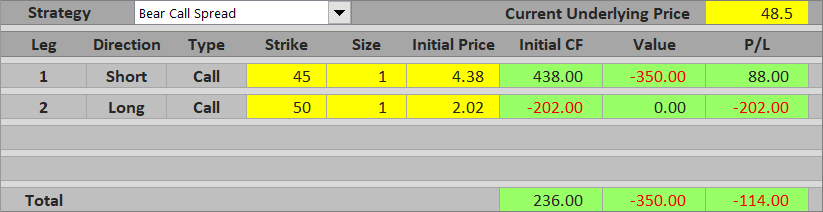Between the two strikes, profit or loss from a bear call spread decreases as underlying price increases. Near the lower strike it approaches maximum profit; near the upper strike it approaches maximum loss.

## Bear Call Spread Payoff Diagram

You can see all the scenarios in the payoff diagram below. The thicker blue line is total P/L, the red line is the short \$45 strike call and the green line is the long \$50 strike call.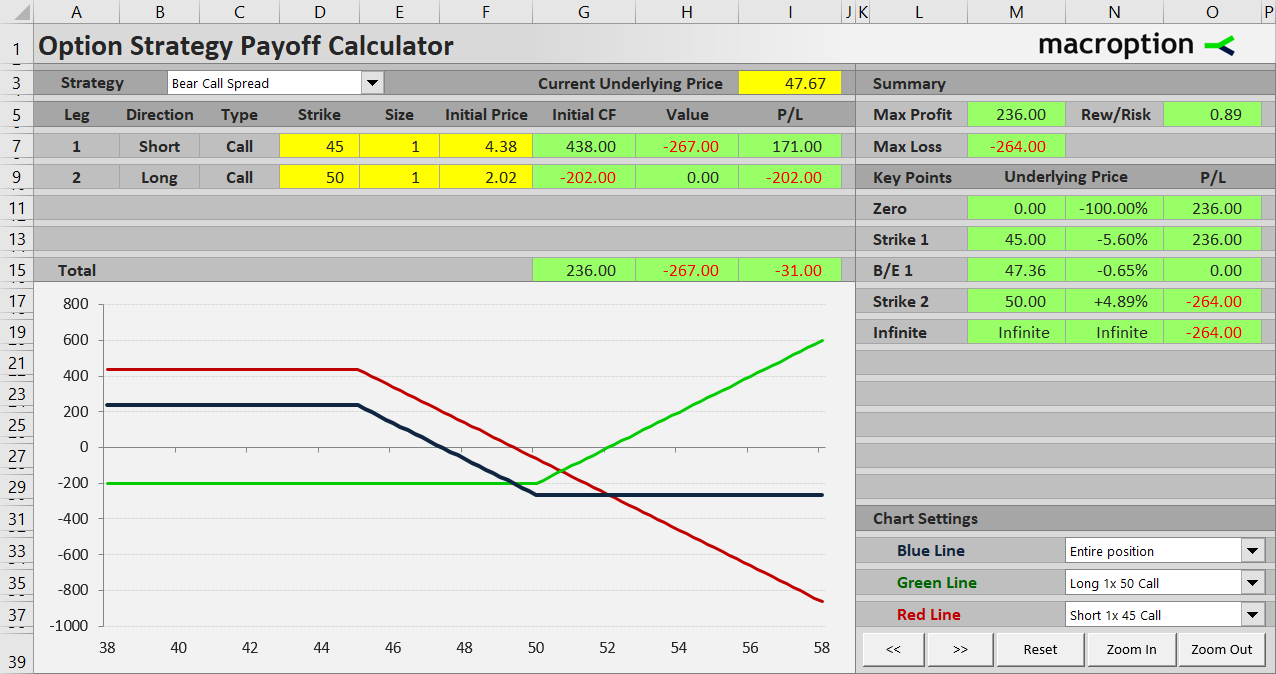Below \$45 the payoff is constant and equal to net premium received – maximum profit.

Between \$45 and \$50 the short call is in the money. As its value increases, the trade's profit declines and eventually turns to a loss. The long \$50 call is still out of the money.

Above \$50 both options are in the money and their further payoff changes exactly offset one another. Total P/L is therefore constant – maximum loss.

## Bear Call Spread Break-Even Point

What is the exact price where the trade turns from profit to loss, or vice versa?

The break-even point is where the value of the short \$45 call is equal to net premium received when opening the position. In our example that is \$236. The \$45 call has this value when underlying price is \$45 + \$2.36 = \$47.36.

General formula for bear call spread break-even point is:

It is exactly the same as bull call spread break-even point, because a bear call spread position is the other side of a bull call spread position (net premium received in the bear call spread B/E formula is really the same thing as initial cost in the bull call spread B/E formula).

## Bear Call Spread Risk-Reward Ratio

To calculate the risk-reward ratio we need to know the risk (maximum possible loss) and reward (maximum possible profit). We already know these from scenarios 1 and 2. For our example, maximum loss is \$264, maximum profit is \$236, and therefore reward is 0.89x risk (R/R = 1:0.89).

The general formulas are as follows:

Maximum loss (risk) = higher strike – lower strike – net premium received

... and also:

Maximum loss (risk) = higher strike – B/E

Comparing bear call spread to bull call spread again, you will find the formulas are exactly the same, just switched (one trade's risk is the other trade's reward and vice versa).

## Similar Option Strategies

Bear call spread has similar payoff profile to bear put spread. The difference is that the latter is made up of puts rather than calls and it is a debit spread (has negative initial cash flow).

Bear call spread can be considered a hedged (more conservative) version of a simple short (naked) call trade. With the long higher strike call you receive less premium and therefore maximum profit from a bear call spread is lower that from a naked call, but you also limit your risk. Distance between the two strikes determines how risky your trade is, as you could see in the maximum loss formula above. The wider the gap between the two strikes, the more a bear call spread's payoff approaches simple short call payoff.

By remaining on this website or using its content, you confirm that you have read and agree with the Terms of Use Agreement.

We are not liable for any damages resulting from using this website. Any information may be inaccurate or incomplete. See full Limitation of Liability.

Content may include affiliate links, which means we may earn commission if you buy on the linked website. See full Affiliate and Referral Disclosure.

We use cookies and similar technology to improve user experience and analyze traffic. See full Cookie Policy.